• ORACLE数据库 DBA常用知识 个系统变量值 SQL> show user --显示当前连接用户 SQL> show error --显示错误 SQL> set heading off ... 开发者的自测利器-Hprof命令（寻找cpu热点） 测试代码: public class HProfTest ...
用vuejs实现一个todolist项目
用vue.js实现一个todolist项目:input输入框输入的值会呈现在下方,并且会保存在localStorage里面,而且下方的列表点击之后也会有变化: 完整代码: App.vue
React&lpar;JSX语法&rpar;-----JSX属性
1. if you know all the propertities that you want to place on a component ahead of time,it is easy t ...
7z压缩文件时排除指定的文件
分享一个7z压缩文件时排除指定文件类型的命令行,感觉很有用: 7z a -t7z d:\updateCRM.7z d:\updateCRM\*.* -r -x!*.log -x!*bak a:创建压缩 ...
ACM 背包问题
背包问题 时间限制:3000 ms  |  内存限制:65535 KB 难度:3   描述 现在有很多物品(它们是可以分割的),我们知道它们每个物品的单位重量的价值v和重量w(1<=v,w&lt ...
转 Java中Filter、Servlet、Listener的学习
1.Filter的功能filter功能,它使用户可以改变一个 request和修改一个response. Filter 不是一个servlet,它不能产生一个response,它能够在一个requ ...
ORACLE数据库 DBA常用知识
<常用命令参考> 个系统变量值 SQL> show user --显示当前连接用户 SQL> show error --显示错误 SQL> set heading off ...
开发者的自测利器-Hprof命令（寻找cpu热点）
测试代码: public class HProfTest { public void slowMethod() { try { Thread.sleep(1000); } catch (Excepti ...
Public&lowbar;1&period;bat
:: targetset targetFileName=Public_2set targetPath=./:: sourceset sourceFileName=Public_1@echo ui ru ...
&lbrack;Day6&rsqb;引用数据类型、ArrayList 集合
1.类的定义与使用 (1)类的定义格式 创建java文件,与类名相同 public class 类名{ 数据类型  属性名称1: 数据类型  属性名称2: … } (2)使用格式 导包:我们将所有的类 ...
【Linux】fg、bg让你的进程在前后台之间切换
Linux下的fg和bg命令是进程的前后台调度命令,即将指定号码(非进程号)的命令进程放到前台或后台运行.比如一个需要长时间运行的命令,我们就希望把它放入后台,这样就不会阻塞当前的操作:而一些服务型的 ...
展开全文• 文章目录关于numpy矩阵运算的小记array与matrix矩阵判等矩阵的连接矩阵的向量化矩阵的拆分矩阵的复制 array与matrix matrix是array的分支，matrix和array在很多时候都是通用的，你用哪一个都一样。但这时候，官方...
关于numpy矩阵运算的小记

文章目录
关于numpy矩阵运算的小记array与matrix矩阵判等矩阵的连接矩阵的向量化矩阵的拆分矩阵的复制矩阵的索引

发现做矩阵处理是numpy忘了好多，所以记录下来.

array与matrix
matrix是array的分支，matrix和array在很多时候都是通用的，你用哪一个都一样。但这时候，官方建议大家如果两个可以通用，那就选择array，因为array更灵活，速度更快，很多人把二维的array也翻译成矩阵。 但是matrix的优势就是相对简单的运算符号，比如两个矩阵相乘，就是用符号*，但是array相乘不能这么用，得用方法.dot() array的优势就是不仅仅表示二维，还能表示3、4、5…维，而且在大部分Python程序里，array也是更常用的。
import numpy as np

a1 = np.array([[1, 2],
[3, 4]])
a2 = np.array([[5, 6],
[7, 8]])
b1 = np.mat([[1, 2],
[3, 4]])
b2 = np.mat([[5, 6],
[7, 8]])

print((np.dot(a1, a2)).all() == (b1 * b2).all())


输出
True
矩阵判等
在矩阵判等中存在各个元素相等和整个矩阵相等两种情况
import numpy as np

a1 = np.array([[1, 2],
[3, 4]])
a2 = np.array([[5, 6],
[7, 8]])
b1 = np.mat([[1, 2],
[3, 4]])
b2 = np.mat([[5, 6],
[7, 8]])
print('np.dot(a1, a2):\n', np.dot(a1, a2))
print('b1 * b2:\n', b1 * b2)
print((np.dot(a1, a2)) == (b1 * b2))
print((np.dot(a1, a2)).all() == (b1 * b2).all())


输出：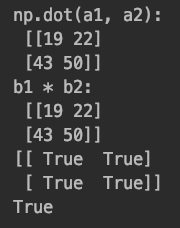其中==用于判等各个元素相等，用all()方法可以判断整个矩阵相等
矩阵的连接
在numpy中存在按行连接与按列连接两种形式
import numpy as np

x = np.array([[1, 2, 3],
[4, 5, 6]])
print("x:\n", x)

y = np.array([[7, 8, 9],
[10, 11, 12]])
print("y:\n", y)

z = np.array([[13, 14, 15]])
print("z:\n", z)

a = np.vstack((x, y, z))
print('np.vstack((x,y,z)):\n', a)
b = np.hstack((x, y))
print('np.hstack((x,y)):\n', b)


输出：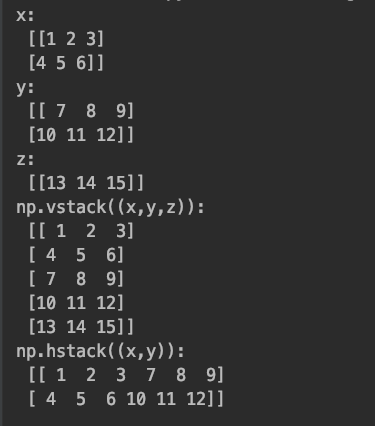其中np.hstack()是按行连接：行数相同的的连接在一起；np.vstack()按列连接：列书相同的连接在一起。
矩阵的向量化
在矩阵中我们可以使用reshape方法来实现矩阵的向量化，如果在整形操作中将尺寸标注为-1，则会自动计算其他尺寸
import numpy as np

x = np.array([[1, 2, 3],
[4, 5, 6]])
print("x:\n", x)

y = np.array([[7, 8, 9],
[10, 11, 12]])
print("y:\n", y)

z = np.array([[13, 14, 15]])
print("z:\n", z)

a = np.vstack((x, y, z))
print('np.vstack((x,y,z)):\n', a)
b = np.hstack((x, y))
print('np.hstack((x,y)):\n', b)

print(a.shape)
print(a.reshape(-1,1))

输出：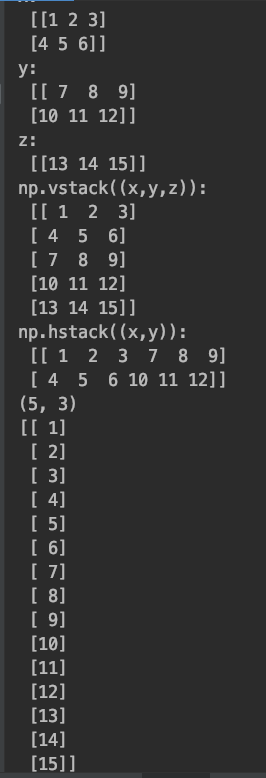矩阵的拆分
使用hsplit，您可以沿数组的水平轴拆分数组，方法是指定要返回的形状相同的数组的数量，或者指定要在其后进行划分的列，使用vsplit，您可以沿数组的竖直轴拆分数组，方法是指定要返回的形状相同的数组的数量，或者指定要在其后进行划分的列。
import numpy as np

a = np.array([[1, 1, 2, 3],
[1, 5, 7, 3],
[7, 3, 9, 3],
[1, 7, 3, 0]])
print(np.hsplit(a, 2))
print(np.vsplit(a, 2))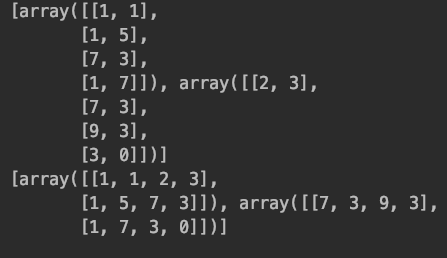矩阵的复制
用=的简单分配不会复制数组对象或其数据，该copy方法对数组及其数据进行完整复制。
import numpy as np

a = np.array([[1, 1, 2, 3],
[1, 5, 7, 3],
[7, 3, 9, 3],
[1, 7, 3, 0]])
# print(np.hsplit(a, 2))
# print(np.vsplit(a, 2))
b = a
print(id(a))
print(id(b))
c = a.copy()
print(id(c))


矩阵的逆
利用numpy.linalg.inv()可以求得矩阵的逆矩阵
import numpy as np

a = np.array([[1, 1, 2, 3],
[1, 5, 7, 3],
[7, 3, 9, 3],
[1, 7, 3, 0]])

b = np.linalg.inv(a)
print(np.dot(a, b))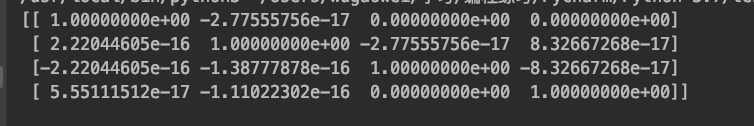矩阵的索引
import numpy as np

a = np.array([[1, 1, 2, 3],
[1, 5, 7, 3],
[7, 3, 9, 3],
[1, 7, 3, 0]])

print(a[2, 3])  # 输出第2+1行第3+1列的数字
print(a[1:])  # 以矩阵形式输出从第1+1行开始的所有数
print(a[1:3])  # 以矩阵形式输出第1+1到第3+1-1行的数
print(a[:, 1])  # 输出矩阵的第1+1列的所有数
print(a[0:3, 1])  # 输出矩阵从0+1行到第3+1-1行的第1+1列的所有数
print(type(a[:3]))

展开全文• # 通过 NumPy可以方便地实现多个矩阵之间的拼接 a = np.random.randint(low=1, high=20, size=(3,3)) print(a) b = np.random.randint(low=1, high=20, size=(3,3)) print(b) #垂直拼接 print(np.vstack((a,b))) #...
import numpy as np

# 通过 NumPy可以方便地实现多个矩阵之间的拼接
a = np.random.randint(low=1, high=20, size=(3,3))
print(a)
b = np.random.randint(low=1, high=20, size=(3,3))
print(b)

#垂直拼接
print(np.vstack((a,b)))

#水平拼接
print(np.hstack((a,b)))

展开全文• numpy 矩阵元素的拼接(concatenate) numpy.append(arr, values, axis=None) 官方文档 示例 Examples >>>np.append([1, 2, 3], [[4, 5, 6], [7, 8, 9]]) array([1, 2, 3, ..., 7, 8, 9]) When axis is ...
接上一期博客
numpy 矩阵元素的拼接(concatenate)
numpy.append(arr, values, axis=None)
官方文档 功能：
Append values to the end of an array.
如果指定了axis，则把arr在axis指定的维度进行切片，并把values拼接在最后. 如果没有指定axis，则把arr和values都做扁平化处理. （要求values在axis指定的维度之外的其他维度和arr有相同的形状）
参数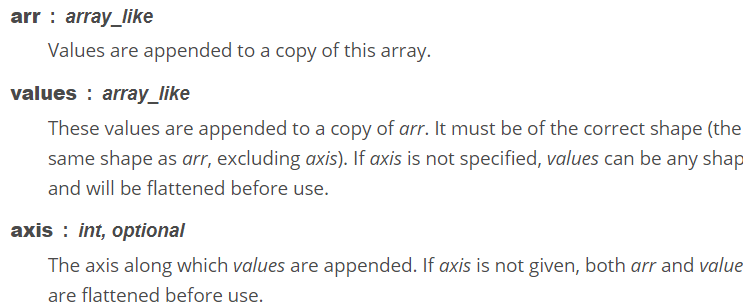示例
>>>np.append([1, 2, 3], [[4, 5, 6], [7, 8, 9]])
array([1, 2, 3, ..., 7, 8, 9])

When axis is specified, values must have the correct shape.
>>>np.append([[1, 2, 3], [4, 5, 6]], [[7, 8, 9]], axis=0)
array([[1, 2, 3],
[4, 5, 6],
[7, 8, 9]])
>>>np.append([[1, 2, 3], [4, 5, 6]], [7, 8, 9], axis=0)
Traceback (most recent call last):
...
ValueError: all the input arrays must have same number of dimensions, but

the array at index 0 has 2 dimension(s) and the array at index 1 has 1 dimension(s)
展开全文python
• 关注微信公众号：一个数据人的自留地作者介绍知乎@王多鱼百度的一...对于数据分析师来说，应掌握基础语法和数据科学的模块，主要包括：pandas、numpy和机器学习库sklearn等。对于算法工程师来说，还应掌握深度学习相...numpy 矩阵乘法 python 空数组
• import numpy as np a = np.array([[1,2,3],[4,5,6],[7,8,9]]) b = np.array([[0,0,0]]) c = np.r_[a,b] d = np.c_[a,b.T] print c print d [[1 2 3] [4 5 6] [7 8 9] [0 0 0]] [[1 2 3 0] [4 5 6 0] [7 ...
• 1、numpy中两个矩阵的合并 1）理论 np.r_[up, down]，把两矩阵上下相加，按列连接两个矩阵，要求列数相等。 np.c_[left, right]，把两矩阵左右相加，按行连接两个矩阵，要求行数相等。 2）例子： import numpy as ...
• 自然有它的强大之处，之前对于特征处理中需要进行数据列表或者矩阵拼接的时候都是自己写的函数来完成的，今天发现一个好玩的函数，不仅好玩，关键性能强大，那就是Numpy模块自带的矩阵、列表连接函数，实践一下。...
• 我有一个Sqlite数据库，其中包含以下类型的架构:termcount(doc_num, term , count)该表包含术语及其在文档中的各自计数。喜欢(doc1 , term1 ,12...我如何使用numpy从稀疏矩阵创建密集矩阵，因为我必须使用余弦相似度...
• python中Numpy库将多个矩阵连接起来的方式横向合并纵向合并合并生成三维矩阵 横向合并 import numpy as np # 随机生成两个4*3矩阵 n1 = np.random.randint(1,10,(4,3)) n2 = np.random.randint(1,10,(4,3)) ...Python
• ## numpy 稀疏矩阵

千次阅读 2017-05-30 13:51:48
scipy.sparse.coo_matrix是三元组，不能按行也不能按列...to_csr 是按行压缩的稀疏矩阵，可以按行切片 to_csc 是按列压缩的稀疏矩阵，可以按列切片 关于压缩存储的格式，见这里http://www.tuicool.com/articles/A3emmqi
• 矩阵拼接方法： np.append(arr,values,axis) np.concatenate(arrays,axis,out=None) np.stack(arrays,axis,out=None) np.hstack/vstack(tup) 下面具体举例，注意输入和输出维度的关系。 1. np.append(arr,values,...
• ## numpy 图像矩阵拼接

千次阅读 2018-12-04 14:48:53图像拼接
• 自然有它的强大之处，之前对于特征处理中需要进行数据列表或者矩阵拼接的时候都是自己写的函数来完成的，今天发现一个好玩的函数，不仅好玩，关键性能强大，那就是Numpy模块自带的矩阵、列表连接函数，实践一下。...
• 自然有它的强大之处，之前对于特征处理中需要进行数据列表或者矩阵拼接的时候都是自己写的函数来完成的，今天发现一个好玩的函数，不仅好玩，关键性能强大，那就是Numpy模块自带的矩阵、列表连接函数，实践一下。...
• numpy 数据(矩阵模块) 种开源的数值计算扩展库.这种库可用来存储和处理大型矩阵,比python自身的嵌套列表结构要高效的多(该结构也可以用来表示矩https://www.runoob.com/numpy/numpy-tutorial.html比较一下 nu....
• 展开全部可以使用concatenate()函数：2113numpy.... : 需要5261拼接的矩阵4102axis : 沿着某个轴拼接，默1653认为列方专向举个例子：属In : import numpy as npIn : a = np.array([[1,2,3],[4,5,6]])In ...
• import numpy as np a=np.array([[1,2,3],[4,5,6]]) b=np.array([[11,12,31],[14,15,16]]) #方法1 c=np.r_[a,b] #沿着矩阵行拼接 print('c=',c) d=np.c_[a,b] #沿着矩阵列拼接 print('d=',d) #方法2 e=np.vstack(...python
• 介绍我非常喜欢Python中的NumPy库。在我的数据科学之旅中，我无数次依赖它来完成各种任务，从基本的数学运算到使用它进行图像分类！简而言之，NumPy是Python中最基本的库之一，也许是其中最有用的库。NumPy高效地...
• 若axis为0表示在第0维将矩阵进行连接，也就是在行上面进行连接连接完的行数等于所有被连接矩阵的行数和 numpy.hstack() 将矩阵按水平方向进行连接，也就是在列上边进行连接连接完的列数等于被连接矩阵的列数和...vstack
• 我试图创建一个混合数据类型(字符串、整数、整数)的numpy数组/矩阵(nx3)。但是当我通过添加一些数据来附加这个矩阵时，会得到一个错误：类型错误：类型提升无效。拜托，有人能帮我解决这个问题吗？当我用示例数据...
• np.r_是按列连接两个矩阵，就是把两矩阵上下相加，要求列数相等，类似于pandas中的concat()。 np.c_是按行连接两个矩阵，就是把两矩阵左右相加，要求行数相等，类似于pandas中的merge()。  import numpy as np a ...
• 矩阵上下拼接 numpy.r_() 矩阵左右拼接 numpy.c_() 矩阵上下拼接 numpy.r_() 类型：numpy模块 语法：numpy.r_(A,B) 参数：1）A ,B均为矩阵，且其列数相同 返回值：矩阵。A,B为具有相同列数矩阵，将...python...